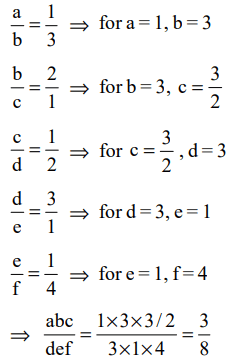## Average, Ratio and Proportion Questions and Answers Part-2

1. A set of consecutive positive integers beginning with 1 is written on the blackboard. A student came along and erased one number. The average of the remaining numbers is $35\frac{7}{17}$  . What was the number erased?
a) 7
b) 8
c) 9
d) None of these

Explanation:2. A change making machine contains 1 rupee, 2 rupee and 5 rupee coins. The total number of coins is 300. The amount is Rs 960. If the number of 1 rupee coins and the number of 2 rupee coins are interchanged, the value comes down by Rs 40. The total number of 5 rupee coins is
a) 100
b) 140
c) 60
d) 150

Explanation:3. At a certain fast food restaurant, Brian can buy 3 burgers, 7 shakes, and one order of fries for Rs 120 exactly. At the same place it would cost Rs 164.5 for 4 burgers, 10 shakes, and one order of fries. How much would it cost for an ordinary meal of one burger, one shake, and one order of fries?
a) Rs 31
b) Rs 41
c) Rs 21
d) Cannot be determined

Explanation: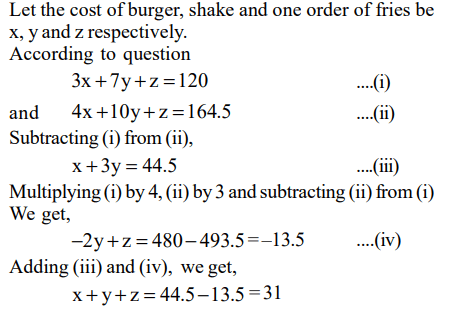4. Mayank, Mirza, Little and Jaspal bought a motorbike for \$60.00. Mayank paid one half of the sum of the amounts paid by the other boys, Mirza paid one third of the sum of the amounts paid by the other boys; and Little paid one fourth of the sum of the amounts paid by the other boys. How much did Jaspal have to pay?
a) 15
b) 13
c) 17
d) None of these

Explanation:5. Using only 2, 5, 10, 25 and 50 paise coins, what will be the minimum number of coins required to pay exactly 78 paise, 69 paise, and Rs 1.01 to three different persons?
a) 19
b) 20
c) 17
d) 18

Explanation: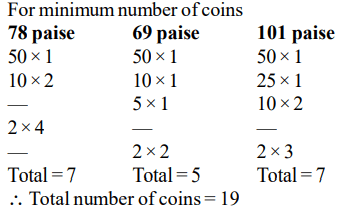6. In a coastal village, every year floods destroy exactly half of the huts. After the flood water recedes, twice the number of huts destroyed are rebuilt. The floods occurred consecutively in the last three years namely 2001, 2002 and 2003. If floods are again expected in 2004, the number of huts expected to be destroyed is
a) Less than the number of huts existing at the beginning of 2001
b) Less than the total number of huts destroyed by floods in 2001 and 2003
c) Less than the total number of huts destroyed by floods in 2002 and 2003
d) More than the total number of huts built in 2001 and 2002

Explanation:7. A milkman mixes 20 litres of water with 80 litres milk. After selling one-fourth of this mixture, he adds water to replenish the quality that he has sold. What is the current proportion of water to milk?
a) 2 : 3
b) 1 : 2
c) 1 : 3
d) 3 : 4

Explanation: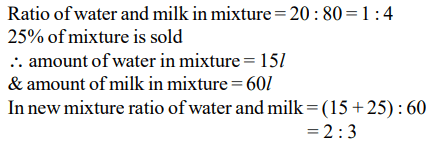8. A sprinter starts running on a circular path of radius r metres. Her average speed (in meters/minute) is $\pi r$ during the first 30 seconds, $\frac{\pi r}{2}$ during next one minute, $\frac{\pi r}{4}$ during next 2 minutes, $\frac{\pi r}{8}$ during next 4 minutes, and so on. What is the ratio of the time taken for the nth round to that for the previous round?
a) 4
b) 8
c) 16
d) 32

Explanation: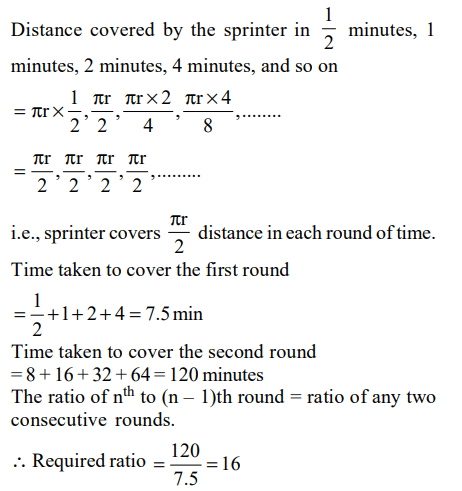9. The number of employees in Obelix Menhir Co. is a prime number and is less than 300. The ratio of the number of employees who are graduates and above, to that of employees who are not, can possibly be:
a) 101 : 88
b) 87 : 100
c) 110 : 111
d) 97 : 8410. If $\frac{a}{b}=\frac{1}{3},\frac{b}{c}=2,\frac{c}{d}=\frac{1}{2},\frac{d}{e}=3$       and $\frac{e}{f}=\frac{1}{4}$  , then what is the value of $\frac{abc}{def}$ ?
a) $\frac{3}{8}$
b) $\frac{27}{8}$
c) $\frac{3}{4}$
d) $\frac{27}{4}$